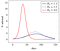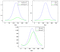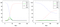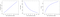# Introduction

The COVID-19 outbreak toolbox contains available, inexpensive and unlimited measures such as social distancing, hygiene and the use of facial masks. On the other hand, there are “finite resources”, the use of which is limited, such as PCR testing, technological systems for “closing the circuit” for detecting and quarantining infected persons through contact-tracing technologies, and quality epidemiological surveillance for quarantining people who have come into contact with verified infected individuals.

These limited resources are inherently expensive and are based on assumptions that only experience over time can calibrate. For example, the “closing the circuit” system is heavily affected by the percentage of asymptomatic infected persons (those who are contagious but will be invisible to the system) and on the extent of civil cooperation which likely might be compromised over time (complacency among the low-risk population and failure to report symptoms). Thus, under certain conditions such as 50% asymptomatic subjects and a low percentage of cooperation, the effectiveness of containment will be low and may even lead to a new outbreak.

The key question in the proposed strategy is how to divide available resources in a way that ensures complete control, over time, until vaccination is obtained, while allowing the economy to continue undisturbed.

The principle proposed is to focus on the part of the population which is sensitive to ”leakage” that is, leakage in the containment of the spread for whatever reason which will lead to flooding of the health system and from there to high mortality rates. Israeli data shows that 84% of those on ventilators have a pre-existing condition (most common: hypertension, diabetes, cardiac) or are over 65 years of age, i.e. a high-risk population. Accordingly:

1. It is desirable to focus most of the expensive resources to the benefit of the high-risk population.
2. However, it is necessary to determine whether leakage in the containment of the spread among the low-risk population will lead to flooding of the health system.

The main innovation in this document is the argument that using the available and unlimited resources of social distancing, hygiene rules, and masks can protect the low-risk population while ensuring that the health system is not flooded. Furthermore, we extend the SEIR model to account for mixed high and low-risk populations with different degrees of containment.

In a paper , the mathematical foundations for bounding the capacity of the health system (i.e., number of respiratory systems) using concentration bounds were developed using statistical confidence intervals and assuming that there is data on the percentage of carriers among the low-risk population by a random sample. In this document, we will use existing data (without the need for a survey) to make a ”back of the envelope” calculation (without confidence intervals) of the maximum number of critical ICU beds (i.e., people on respiratory systems) required to contain the peak of the outbreak from the low-risk population, given an exponential spread of R_0= 1.4 according to the SEIR model (a detailed description of the model deferred to Appendix A). That is, an infected person infects on average 1.4 people. We chose to set the ”basic reproduction number” R_0 to 1.4 as it is in the low range of R_0reported in the literature . The reason for taking the low-range of R_0 is that we would consider releasing the low-risk population from quarantine under social-distancing guidelines, hygiene, facial masks and restrictions of gatherings above certain thresholds. Later on, we will tackle the problem of modeling potential leakage from the low-risk population to the high-risk population.

The graph below shows the percentage of infectious persons according to the SEIR model (see captions for details). As can be seen, the curve has a “bell-shape”, and the largest load on the health system will happen at the peak of the curve. For R_0 = 1.4, at the peak of the curve we have 1.78% infectious persons. Multiplying this by the probability of an infected person from the low-risk population needing a ventilator, and by the ratio between the average ventilation time (11 days) and average infection time (2.9), we can obtain the percentage of the low-risk population that will need a ventilator at the peak of the outburst.Figure 1: The percentage of infectious people as a function of time according to the SEIR model (see Appendix A), with the parameters τi = 2.9; τe = 5 and several options for the R₀ parameter.

The probability of needing ventilation can be calculated from existing data: As of 7th April, 2020, there were 19 ventilated infected persons in Israel from the low-risk population (out of a total of 116). Ten days earlier, there were around 5,000 verified infected persons. Clearly, a lower bound on the number of infected persons would be twice the number of verified infected persons due to the fact that around 50% of infected people are without symptoms . Furthermore, a recent survey in Austria  found that the true number of infected persons is two to five times the number of verified infected persons. Taking the worst case of a factor of two, suppose the true number of infected individuals was 10,000. From the analysis of the age distribution of infected persons, one may conclude that 15% are over 65. Suppose there is another 10% of infected persons below the age of 65 but with acute background diseases. Therefore, in the absence of data with a confidence interval from a representative survey, we discern from existing information that: Probability of ventilation in low-risk population ≈ 19/7500 = 0.0025. Note that the probability of requiring ventilation given infected is a fixed number (to a particular population) regardless of the time the estimation was made.

The size of the low-risk population is approximately 7 million people (8.8 million minus 1.2 million over 65 minus 600,000 people with acute background diseases).

The number of ventilated at the peak of the outbreak is therefore

It is not inevitable that the actual number of infected persons is significantly greater than factor two of verified infected. As mentioned above, according to the Austrian survey, the factor can reach as much as five times, i.e., the number of those on ventilators at the peak of the outbreak will range from 472 to 1,181. Thus, even with the worst-case assumptions, preparation for ≈1200 ventilated infected persons may be easily arranged considering the ability of the existing healthcare system and procurement mechanisms in Israel.

In addition, it is easy to see that the “leakage” of the spread of the disease in the low-risk population does not translate into an unmanageable increase in the number of those on ventilators. For example, suppose the basic reproduction number R_0 is 1.5 (“leakage” from 1.4), then, according to the SEIR model, the number of needed ventilators will increase to about 1,600.

It is important to note that accurate data and estimates can be obtained by a sample survey that will almost certainly alleviate the worst-case assumptions adopted in estimating the true number of infected persons in relation to the verified infected persons.

The conclusion from which operative measures can be derived is that the sensitivity to leakage in the low-risk population is dramatically lower than the sensitivity to leakage in the high-risk population. Moreover, the State of Israel will be able to prepare for the maximum number of ventilated patients under the worst-case assumptions adopted.

So far, we have ignored the high-risk population. In reality, however, there will be some leakage from infectious people in the low-risk population to the high-risk population and leakages among the high-risk population. In Appendix B we generalize the SEIR model so that it will take into account a division to two populations. This is modeled by four values of the basic reproduction number: R_0l,l ;R_0l,h ; R_0h,l ; R_0h,h, representing low-to-low, low-to-high, high-to-low, and high-to-high infections.

As above, we set R_0l,l = 1.4 which represents the spread of the virus among the low-risk population to an infected person infecting (on average) 1.4 persons. The leakage from low to high groups, R_0l,h is assumed to be small because both populations understand the risks and we set it to 0.02 (meaning that on average 50 infected people from the low group will infect one person from the high group). For example, in Israel people are told ”stay away from your grandparents” and this, by and large, is being self-imposed across the country. The leakage from high to low is not material to our model but for the sake of symmetry, we set it to R_0h,l = 0.02 as well. What remains is to set reasonable expectations to the possible leakage among the high-risk population. Given that (i) the population is under stricter social distancing, and (ii) by and large the group is heavily tilted towards senior citizen ages where the percentage of single-person household is heightened, then it is reasonably to assume that R_0h,h < 1 and we set it to 0.7. The results are displayed in Figure 2 showing that the added number of critical ICU beds (on top of the 1181 for the low-risk group) stands on 872 (under pessimistic assumptions).

Taken together, taking into accounts leaks between the two risk groups and among the high-risk group, with a worst-case assumption of factor 2 between the validated positives and true positives, the total number of critical ICU beds needed at the peak of the outbreak stands on 2,053 — which translates to 20 critical beds per 100,000 inhabitants.

The operative proposal does not change the basket of means and resources but rather their focus. Due to the low sensitivity to leakage in the low risk population, the lockdown upon that population may be released subject to social distancing guidelines, hygiene rules and masks. At the same time, it will be possible to restore the economy and the education system to routine, while the retail, leisure and restaurant sectors may be restored subject to restrictions. The high tolerance to leakage allows for great flexibility in the “dosage” of lockdown release.

At the same time, the high-risk population will be managed according to stricter social distancing guidelines, with ”closing the circuit” resources directed primarily at this population and those treating them (nursing homes, hospitals, etc.). Among this population, the leakage sensitivity is high (with a risk of flooding the health system) and therefore, it is desirable that the expensive and limited resources be diverted in greater measure to the prevention of leakage in the spread of the virus among high-risk individuals. By monitoring the seriously ill in this population, one may control the level of intensity of the guidelines and social distancing rules.

As for mortality, it is important to note that, by segregation according to risk groups as suggested here, total mortality across the general population will be lower compared to methods that apply across the general population. The alternative is an ongoing lockdown, or ongoing rounds of lockdown and release depending on the effectiveness of ”closing the circuit” among the entire population. As mentioned, the success of ”closing the circuit” critically relies on the cooperation of the population and it is safer to assume that those who in the high-risk group would be more cooperative in reporting symptoms as soon as they arise.

A back-of-the-envelope estimation of mortality of the low-risk group (which under this proposal are consciously subjected to the risk of infection), to date there is zero mortality among the low-risk group in Israel. Given that there were 19 individuals using ventilators a lower-bound on the probability of mortality is 1/19 the probability to require a ventilator, i.e., 0.0025/19 = 0.00013 which is 0.013% of the low-risk population. Given R_0 = 1.4 the total number of people infected (over a period of roughly 200 days) is around 50% (see Figure 4 right-most panel). This translates to a figure of 455 under the worst case assumption of factor 2 between the validated positives and true positives (if the factor is larger, say factor 5, then the mortality figure would be 182). For the sake of calibration, the annual mortality in Israel due to traffic accidents stands around 350.

# Appendix A: The SEIR model

The Susceptible-Exposed-Infected-Recovered (SEIR) model is a simple compartmental mathematical model of infectious disease. In this model, the total population is divided into S susceptible persons (people that did not get the disease yet), E exposed persons (people that has been exposed but are still not ill), I infected persons (people that has been infected and can infect others), and R recovered persons (people that may be recovered/dead/or still be sick, but are not infectious any more). The total population size is the sum N = S + E + I + R. Every day, the relative sizes of the different compartments change. We denote by S[t], E[t], I[t], R[t] the number of persons in each compartment on day t. The update of the sizes is according to the following rules:

• Let τᵢ be the average number of days a person can infect others. Recall that in our model, the Recovered compartment includes people that can no longer infect others (either because they recovered, or because they died, or because they are still sick but are no longer infectious). Therefore, every day, a fraction of 1/τᵢ of the Infected compartment is moved to the Recovered compartment. This yields:
• Let τₑ be the average number of days from the moment a person is exposed to the virus until he becomes infectious. Then, every day, a fraction of 1/τₑ of the Exposed compartment is moved to the Infected compartment. This yields:
• The basic reporduction number, denoted R_0, is the average number of persons an infected person will expose in total, if he will just meet persons from the S compartment. The probability to meet a person, particularly from the S compartment on day t, is S[t]/N. Therefore, the total number of persons an infected person will expose on day t is R_S[t]/N. Spreading this over τi days of infection, we get that on every day, each person from the I compartment exposes R_0 · (S[t]/N) · (1/τᵢ) persons. It follows that:
• and,Figure 2: The mixed SEIR model with the parameters τi = 2.9, τe = 5, R0l,l = 1.4, R0h,h = 0,7, R0l,h = R0h,l=0.02, N^l = 7,000,000, N^h=2,000,000. The bottom graph is an estimation of the number of severe cases based on pessimistic statistics of 4%; 0:25% probabilities to be severe in the high/low-risk groups respectively. These estimates are based on the same calculations as in Section 2.

To get some intuition, the graphs below depict the values of S[t]/N, E[t]/N, I[t]/N, R[t]/N, where we set τᵢ=2.9, τₑ = 5, R_0 = 3.0.

As can be seen, the Susceptible compartment monotonically decreasing and the Recovered compartment is monotonically increasing. The Infected and Exposed compartments have a “bell-shape”, where initially they are growing fast and then they decay fast. The basic reproduction number, R_0, determines the “flatness” of the bell-shape: what is the height at the peak and on which day we arrive at the peak. The basic reproduction number also determines the size of the Recovered compartment at the end of the pandemic (how many people got sick sometime in the past and are now either recovered or died). The graphs below depict the height of the peak, day at the peak, and the recovered compartment at the end of the pandemic, as a function of R_0.Figure 3: SEIR model with the parameters τi = 2.9; τe = 5. Left R0 = 3.0, Right: R0 = 1.5.Figure 4: The effect of the basic reproduction number.

The SIR model: In the pure SIR model, there are only three compartments: Susceptible, Infected, and Recovered. The formulas are similar

The derivative (change) of I[t] is I[t + 1]-I[t] = (R_0S[t]/(Nτi)-(1/τi)) I[t]. When the derivative of I[t] equals to zero, we reached the peak of the pandemic, and from there on, the number of infected persons will decrease over time. Based on the above expression, this will happen when S[t]/N=1=R₀. Denoting the portion of the population which is not susceptible by φ[t] := 1 — S[t]/N, then we obtain that the peak of the pandemic is when φ[t] = 1–(1/R_0). Note that this formula is incorrect for the SEIR model. And, in any case, we do not need to rely on the formula as we are simulating the entire dynamic.

# Appendix B: A SEIR model with a division to High and Low-Risk Populations

We now describe a variant of the SEIR model, in which there are two populations: those of high risk and those of low risk. As in the SEIR model, each sub-population is divided into the four compartments of Susceptible, Exposed, Infectious, and Recovered. We denote the 8 compartments at time t by Sˡ[t], Eˡ[t], Iˡ[t], Rˡ[t], Sʰ[t], Eʰ[t], Iʰ[t], Rʰ[t], where the upper script l or h designates low or high risk groups. We assume mild social distancing within the low-risk group and strict social distancing between the low and high risk groups as well as within the high risk group. This is modeled by four values of the basic reproduction number: R_0l,l ; R_0l,h ; R_0h,l ; R_0h,h, representing low-to-low, low-to-high, high-to-low, and high-to-high infections. The update equations for Iˡ, Iʰ, Rˡ, Rʰ are similar to the vanilla SEIR model. As to the move from suspects to exposed: each person in potentially infects R_0l,l/τi persons in one day if he happens to meet someone from Sˡ (which will happen with probability Sˡ/ where is the size of the low-risk population), and in addition, potentially infects R_0l,h/τi persons in one day if he happens to meet someone from (with probability Sʰ/Nʰ). Similarly for each person in Ih. This gives the following difference equations:

Figure 2 (top) shows the percentage and number of infected cases for the two populations. The parameters we used are where we set τi = 2.9; τe = 5, R_0l,l = 1.4, R_0h,h = 0.7, R_0l,h = R_0h,l = 0.02, = 7,000,000, = 2,000,000. The rationale for the parameters is that the low-risk group has mild social distancing measures while the high-risk group has strict social distancing measures, and in particular, any contact between the two groups must be with significant protection (masks, gloves, etc.).

 T. John. Iceland lab’s testing suggests 50% of coronavirus cases have no symptoms. https://edition.cnn.com/2020/04/01/europe/iceland-testing-coronavirus-intl/index.html.
 Q. Li, X. Guan, P. Wu, X. Wang, L. Zhou, Y. Tong, R. Ren, K. S. Leung, E. H. Lau, J. Y. Wong, et al. Early transmission dynamics in wuhan, china, of novel coronavirus–infected pneumonia. New England Journal of Medicine, 2020.
 A. Shashua and S. Shalev-Shwartz. Can we contain covid-19 without locking-down the economy? CBMM Memo 104, Massachusetts Institute of Technology, 2020.

 COVID-19 Prevalence, SORA, April 10, 2020. https://www.sora.at/uploads/media/Austria_COVID19_Prevalence_BMBWF_SORA_20200410_EN_Version.pdf

CEO of Mobileye, SVP at Intel, Co-CEO of OrCam, Chairman of AI21labs & Sachs Prof. of Computer Science at the Hebrew University of Jerusalem

CEO of Mobileye, SVP at Intel, Co-CEO of OrCam, Chairman of AI21labs & Sachs Prof. of Computer Science at the Hebrew University of Jerusalem